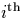Next: Signed Binary Arithmetic Up: Logistic Map Previous: Logistic Map

Suppose we restrict ourselves to dyadic streams. In order to obtain n digits of f(x), we compute (1-x) which has a lookahead of (n+1) (because the subtraction operation uses a shift), multiply this by x which gives (n+2), and shift the result left to achieve multiplication by 4. This means the function will require (n+4) lookahead. If this is now iterated, at the second iteration we will require (n+4) digits of f(x) which is ((n+4)+4) digits of x. Hence at theiteration the lookahead will be (n+4i).